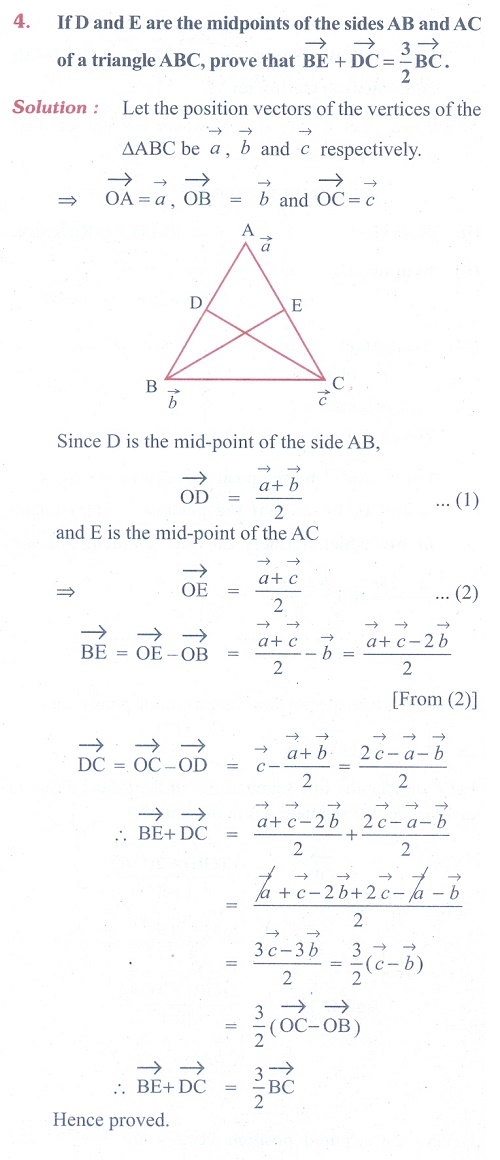Home | | Maths 11th std | Exercise 8.1: Position vectors

# Exercise 8.1: Position vectors

Maths Book back answers and solution for Exercise questions - Mathematics : Vector Algebra: Position vectorsTags : Problem Questions with Answer, Solution | Mathematics , 11th Mathematics : UNIT 8 : Vector Algebra I
Study Material, Lecturing Notes, Assignment, Reference, Wiki description explanation, brief detail
11th Mathematics : UNIT 8 : Vector Algebra I : Exercise 8.1: Position vectors | Problem Questions with Answer, Solution | Mathematics